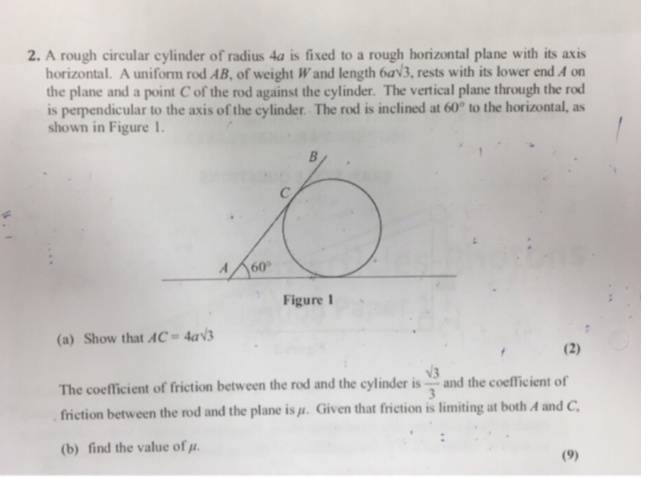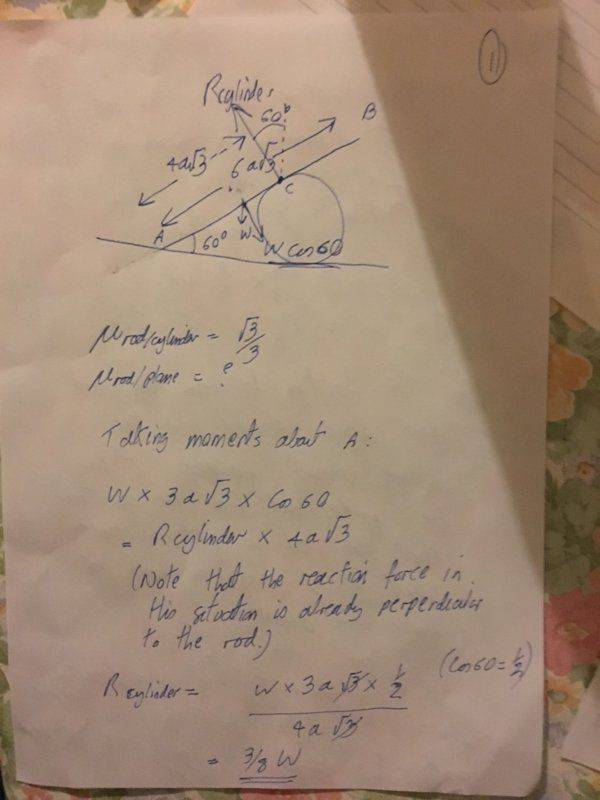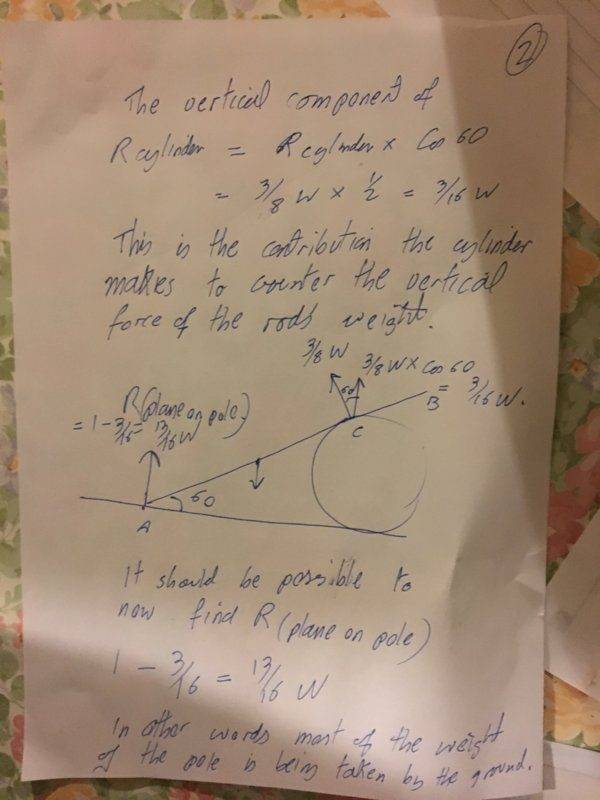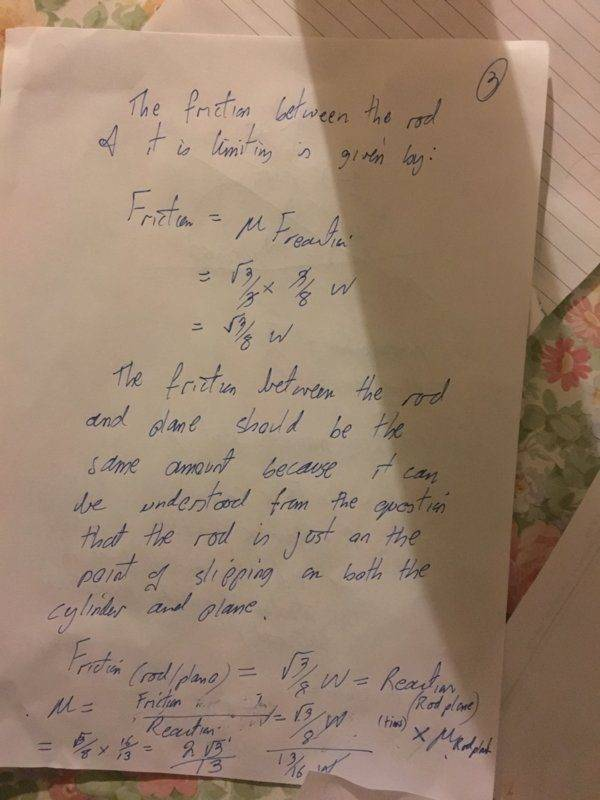# Rod resting on a cylinder, find mu

• resurgance2001
resurgance2001
Homework Statement
Please see the photo of the question below with the diagram. We have to find the coefficient of friction between the rod and plane
Relevant Equations
Moments, Components of forces, and friction = mu x reaction force.
My solution is on the files.

The basic approac was the take moments about A. Then find the reaction at C.

Use the reaction at C to find the friction between rod and cylinder.

I take it to mean from the question that the friction between the rod and plane is equal to the friction between the rod and cylinder.

So find reaction at A.

And then divide the friction by the reaction at A.

So my real question is do you agree with the approach or can you suggest a more elegant way of solving the problem. ?

ThanksHomework Helper
Gold Member
I do not agree with your method. There are additional forces ##F_x## and ##F_y## at the point where the rod touches the ground. You need to take these into account and write three equations balancing the horizontal forces, the vertical forces and the torques.
I take it to mean from the question that the friction between the rod and plane is equal to the friction between the rod and cylinder.
The statement "Given that friction is limiting at both A and C" does not mean that the forces of friction are equal; it means that at both A and C the rod is at the threshold of slipping.

I would use a coordinate system with vertical and horizontal axes otherwise you will go crazy trying to sort out the force of static friction where the rod meets the ground.

resurgance2001
I do not agree with your method. There are additional forces ##F_x## and ##F_y## at the point where the rod touches the ground. You need to take these into account and write three equations balancing the horizontal forces, the vertical forces and the torques.

The statement "Given that friction is limiting at both A and C" does not mean that the forces of friction are equal; it means that at both A and C the rod is at the threshold of slipping.

I would use a coordinate system with vertical and horizontal axes otherwise you will go crazy trying to sort out the force of static friction where the rod meets the ground.
Thanks. I have another answer that someone else has done. It looks like they are doing the right thing with what looks like possibly a good free body diagram. However, at the start of their answer they just seem to pull out of nowhere 1/2 NRC - I can’t see what the justification is for that equation. Are they balancing moments. The diagram looks convincing but I am not convinced it is the correct answer.